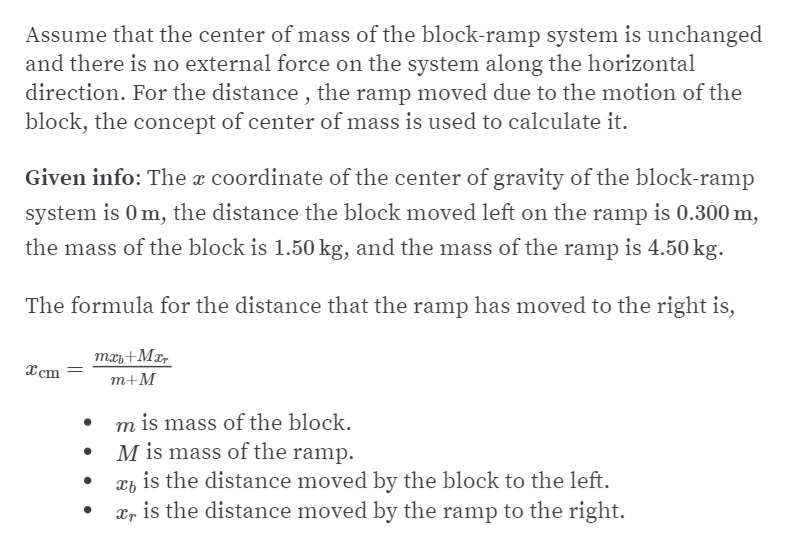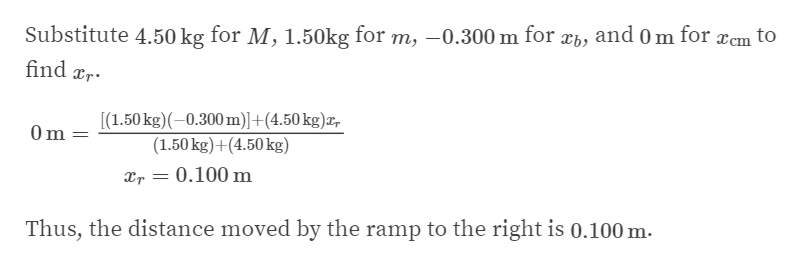Question
1 views

A block of mass m = 1.50 kg is at rest on a ramp of mass M=
4.50 kg which, in turn, is at rest on a frictionless horizontal
surface (Fig. P8.13a). The block and the ramp are aligned
so that each has its center of mass located at x = 0. When
released, the block slides down the ramp to the left and the
ramp, also free to slide on the frictionless surface, slides to
the right as in Figure P8.13b. Calculate xramp, the distance the
ramp has moved to the right, when xblock = -0.300 m.

check_circle

Step 1

Here,help_outlineImage TranscriptioncloseAssume that the center of mass of the block-ramp system is unchanged and there is no external force on the system along the horizontal direction. For the distance , the ramp moved due to the motion of the block, the concept of center of mass is used to calculate it. Given info: The x coordinate of the center of gravity of the block-ramp system is 0 m, the distance the block moved left on the ramp is 0.300 m, the mass of the block is 1.50 kg, and the mass of the ramp is 4.50 kg. The formula for the distance that the ramp has moved to the right is, тль+ Mz, Xcm т+M m is mass of the block. M is mass of the ramp. is the distance moved by the block to the left. xr is the distance moved by the ramp to the right. fullscreen
Step 2

Thus,&nbs...help_outlineImage TranscriptioncloseSubstitute 4.50 kg for M, 1.50kg for m, –0.300 m for xb, and 0 m for æcm to find rr. [(1.50 kg)(-0.300 m)]+(4.50 kg)x, (1.50 kg)+(4.50 kg) 0m 0.100 m Thus, the distance moved by the ramp to the right is 0.100 m. fullscreen

### Want to see the full answer?

See Solution

#### Want to see this answer and more?

Solutions are written by subject experts who are available 24/7. Questions are typically answered within 1 hour.*

See Solution
*Response times may vary by subject and question.
Tagged in

### Rotational Mechanics